# 写在前面

1. ACM 训练（复习）的时候重新学习了一下常见的 DP 转移的优化技巧，在学习的同时也有一些自己的理解，便一并总结在这。
2. 本文成文前阅读或参照了许多大佬的博客，这些将附在文末参考文献中。
3. 若文章中出现错误，烦请告知。感谢您的造访。

# 决策单调性对一类 1D/1D DP 的优化

$$f_i = \min(\max){f_j+w(i,j)}$$

## $w(i,j)$ 只含 $i$ 和 $j$ 的项——单调队列优化

### 单调队列优化多重背包

for (int i = 1; i <= n; i++)
for (int k = 1; k <= c[i]; k++)
for (int j = V; j >= v[i]; j--)
f[j] = max(f[j], f[j-v[i]]+w[i]);

## $w(i,j)$ 只含 $i,j$ 和 $ij$ 的项——斜率优化

$$-d[j]=a[i]\times b[j]+c[i]-f_i$$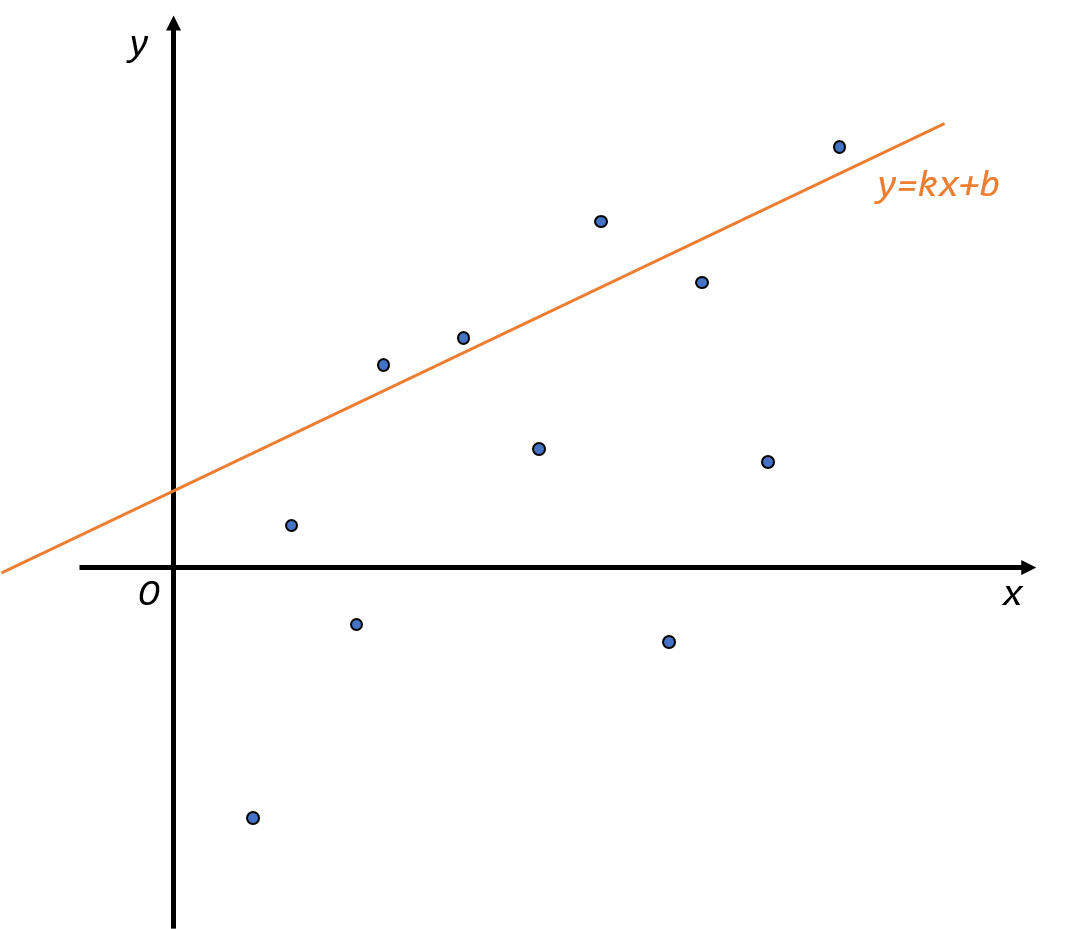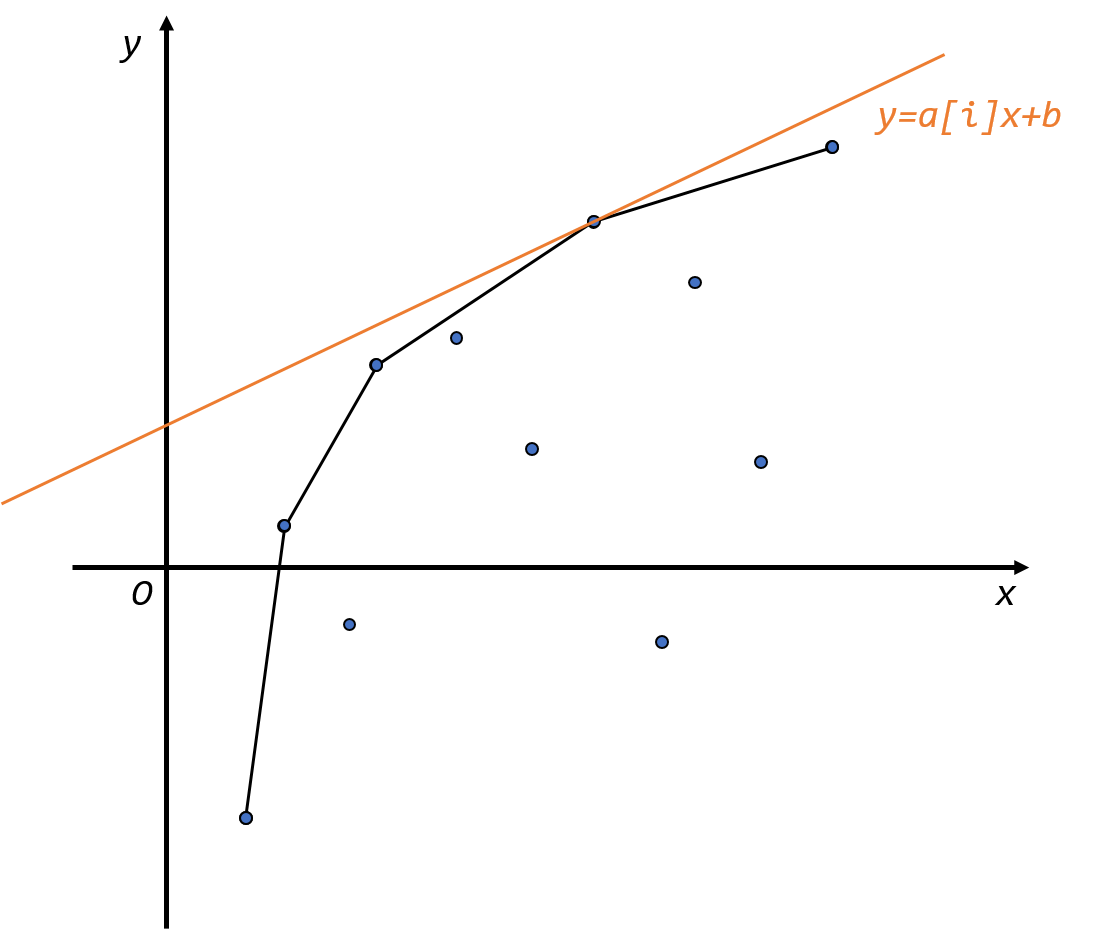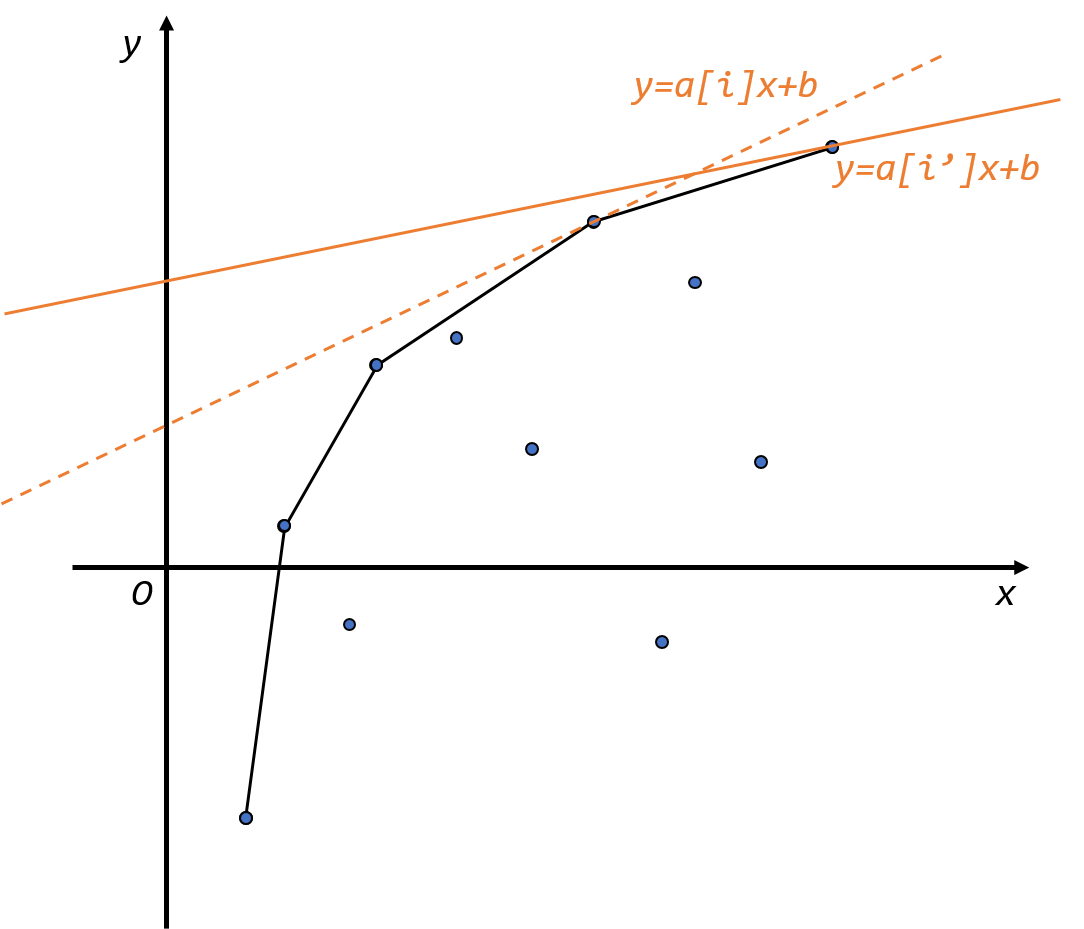## 决策单调性适用的原理——四边形不等式与决策单调性

• 当四边形不等式不等号取 $\geq$ 时，若转移方程是取 $\min$ 值，那么最优决策点与 $i$ 的移动方向相，并且单调；
• 当四边形不等式不等号取 $\geq$ 时，若转移方程是取 $\max$ 值，那么最优决策点与 $i$ 的移动方向相，并且单调；
• 当四边形不等式不等号取 $\leq$ 时，若转移方程是取 $\min$ 值，那么最优决策点与 $i$ 的移动方向相，并且单调；
• 当四边形不等式不等号取 $\leq$ 时，若转移方程是取 $\max$ 值，那么最优决策点与 $i$ 的移动方向相，并且单调。

$$f_{p_i}+w(p_i,i)\leq f_j+w(j,i)\tag{1}$$

$$f_{p_{i+1}}+w(p_{i+1},i+1)\leq f_j+w(j,i+1)\tag{2}$$

$$f_{p_{i+1}}+w(p_{i+1},i+1)\leq f_{p_i}+w(p_i,i+1)\tag{3}$$

1. $p_{i+1}=i$，那么显然 $p_{i+1}=i\geq p_i$ 的，满足决策单调性；
2. $p_{i+1}\neq i$，将 $(1)$ 式中 $j$ 变为 $p_{i+1}$，那么
$$f_{p_i}+w(p_i,i)\leq f_{p_{i+1}}+w(p_{i+1},i)\tag{4}$$
$(3)+(4)$ 得
$$w(p_{i+1},i+1)+w(p_i,i)\leq w(p_i,i+1)+w(p_{i+1},i)$$

\begin{aligned} w(i,q)+w(j,p)&=a[i]+b[q]+a[j]+b[p]\ w(i,p)+w(j,q)&=a[i]+b[p]+a[j]+b[q] \end{aligned}

\begin{aligned} w(i,q)+w(j,p)&=a[i]\times b[q]+c[i]+d[q]+a[j]\times b[p]+c[j]+d[p]\ w(i,p)+w(j,q)&=a[i]\times b[p]+c[i]+d[p]+a[j]\times b[q]+c[j]+d[q] \end{aligned}

$$a[i]\times b[q]+a[j]\times b[p]\geq a[i]\times b[p]+a[j]\times b[q]$$

• 当 $w$ 符号取 $\geq$，DP 取 $\min(\max)$ 值时，$a$ 与 $b$ 的单调性相反即可，只不过要根据决策点向左/向右移动来选择单调栈/单调队列维护。
• 当 $w$ 符号取 $\leq$，DP 取 $\min(\max)$ 值时，$a$ 与 $b$ 的单调性相同即可，同上。

## $w(i,j)$ 满足四边形不等式的更一般的情形——分治/二分数据结构

### 分治

$$f_i = \min(\max){g_j+w(i,j)}$$

### 二分+数据结构

$$f_i = \min(\max){f_j+w(i,j)}$$

$$w(m,j)+w(n,i)\geq w(m,i)+w(n,j)\tag{1}$$

$$f_m+w(m,i)\geq f_n+w(n,i)\tag{2}$$
$(1)+(2)$ 得
$$f_m+w(m,j)\geq f_n+w(n,j)$$

00112222234444666

00000000000000000

00111111111111111

1. 队尾区间未被完全覆盖，这个时候显然二分出最左端的更新位置之后，把当前队尾的右端点更新，再把新的这个三元组加进队尾。
2. 新的元素不比任何一个更优，我们只需要在二分前做一次特判，看新的元素更新 $n$ 这个位置是否比之前的更优，若否，那么显然这个元素不可能成为后续的最优决策，舍掉。
3. 队尾区间能被完全覆盖，也就是说，例如原来的最优决策点是
00112222234444666

新加入的 7 这个点能完整地覆盖 6，如
00112222234477777

那么这时，我们只需在二分前把这种能被完全覆盖的区间去掉就可以了，操作方法是比较队尾元素作为决策点以及新的元素作为决策点转移到队尾元素控制的左端点哪一个更优，若新的元素能更优，把队尾弹出。重复操作后就可以按照 1. 的情形二分了。

1. 二分的区间的左端点是经过第三种情形处理后的队尾元素的左端点，二分的右端点是 $n$。
2. 情形 3 是可以边二分边弹出队尾的。不过若这样做，一定要注意计算 DP 值的时候千万不要用后面的元素视作前面元素的决策。几个例子
00112222234444666

当你计算 4 这个区间的 DP 值时，千万不要拿 6 作为最优决策点来算，因为这样就不满足决策单调性了。所以还是建议先弹出不合法的队尾再进行二分。
3. 对于每个位置 $i$，它的最优决策点就是队首元素，不过如果队首元素控制范围的右端点在 $i$ 前面，记得把这个队首弹出再取新的队首作为最优决策点。

• $f$ 与 $g$ 无关，或者与此同时 $w$ 不能简易计算出，选择分治法；
• $w$ 能简易计算出，选择二分+数据结构的方法。

# 四边形不等式对 2D/1D DP 的单调性优化

• 四边形不等式，还是以 $\forall a,b,c,d, a\leq b\leq c\leq d, w(a,d)+w(b,c)\geq w(a,c)+w(b,d)$ 为例。
• 区间单调性，即 $\forall a,b,c,d, a\leq b\leq c\leq d, w(a,d)\geq w(b,c)$，即小区间的函数值不超过包含其的大区间。并且式中的不等号应时刻与四边形不等式的不等号方向一致。

$l=1$，$(l,l+\delta-1)$ 枚举长度是 $s_{l+1,l+\delta-1}-s_{l,l+\delta-2}=s_{2,\delta}-s_{1,\delta-1}$；
$l=2$，枚举长度是 $s_{3,1+\delta}-s_{2,\delta}$；
$l=3$，枚举长度是 $s_{4,2+\delta}-s_{3,1+\delta}$；
$\vdots$
$l=n+1-\delta$，枚举长度是 $s_{n+2-\delta,n}-s_{n+1-\delta,n-1}$。

# 不满足决策单调性的斜率优化

1. 随着 $i$ 往后枚举，平面上新加入的点均出现在已有的点集的右（或左）侧，这样新加入的点一定会在凸包上。因此可以比较加入的点和凸包边缘的点的关系，维护凸包。整个过程复杂度可以做到 $O(n)$；
2. 随着 $i$ 往后枚举，$i$ 这一点对应的线性规划的直线的斜率是单调的。同时单调数据结构维护的凸包上的点两两相邻的斜率也是单调的，因此如果队首的点不再是 $i$ 点的最优决策点的话，那么可以删掉队首，因为队首不再可能是之后的最优决策点。同样，这个过程复杂度也为 $O(n)$。

## 加入点横坐标不单调——平衡树维护凸包/CDQ 分治

1. 先递归处理 $[l, mid]$ 这一区间；
2. 对 $[l, mid]$ 区间内所有点按横坐标排序，用决策单调性的方法维护这一区间内的上（或下）凸包；
3. 之后把 $[mid+1, r]$ 区间内所有的点按询问的直线斜率排序，同样用决策单调性的方法去询问；
4. 递归处理 $[mid+1, r]$ 区间。

# 带权二分优化（DP 凸优化）

• [BZOJ 2654]tree(陈立杰)

给你一个 $n$ 个点 $m$ 条边的无向带权连通图，每条边是黑色或白色。让你求一棵最小权的恰好有 $need$ 条白色边的生成树。
$1\leq n\leq 50000, 1\leq m\leq 100000$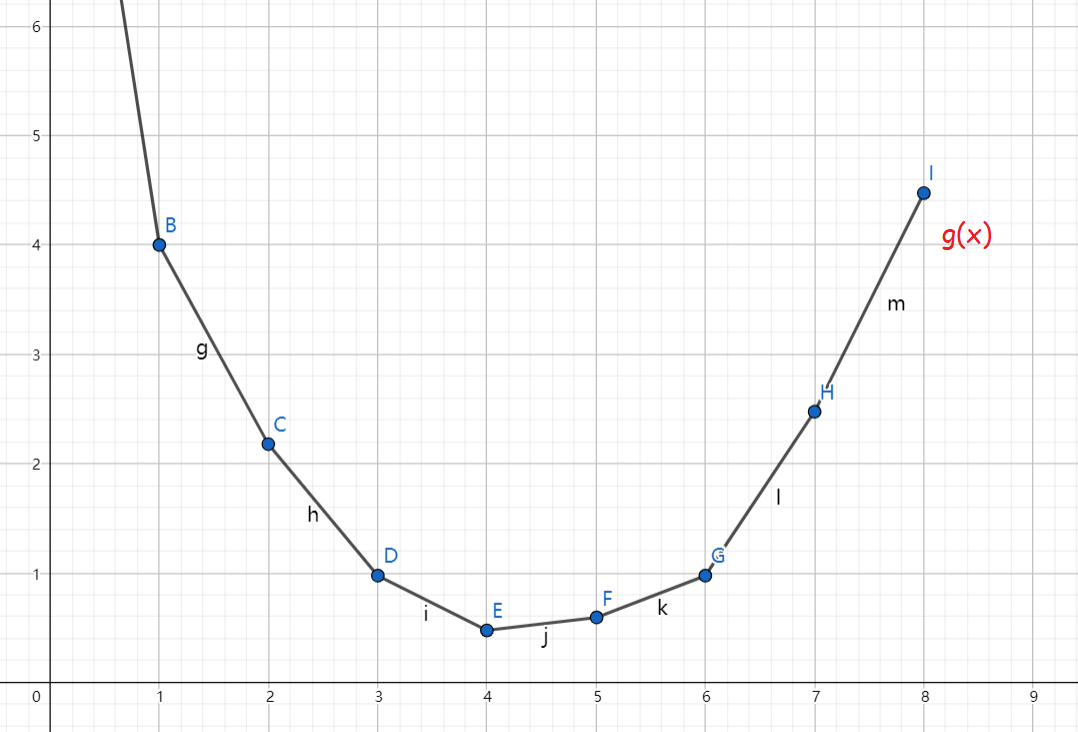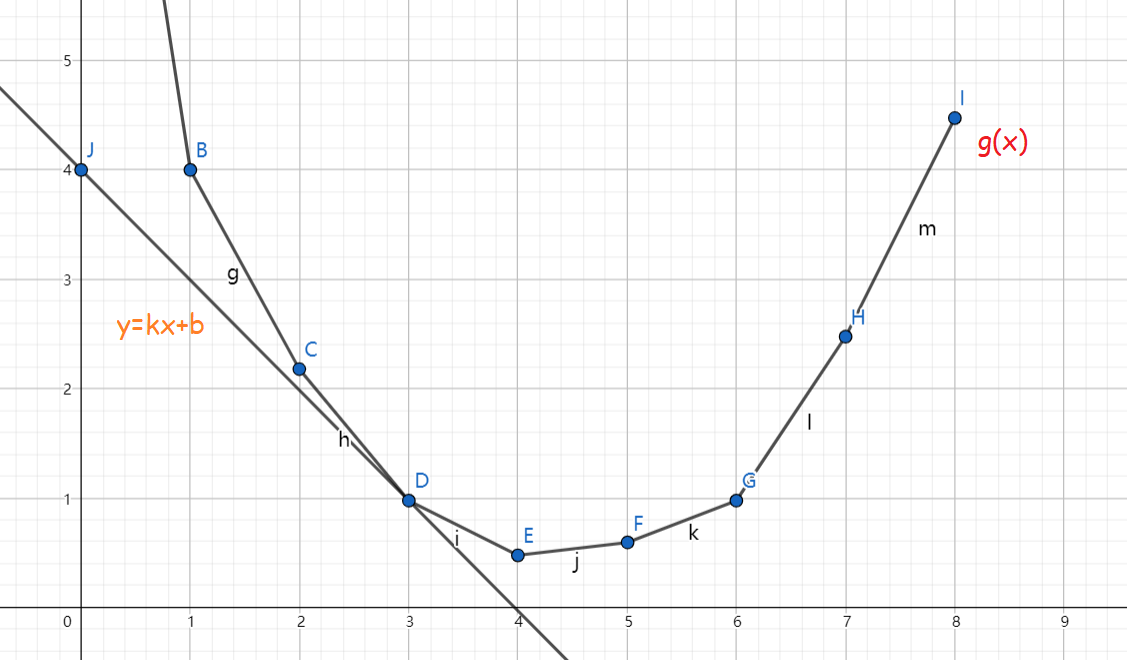• 二分值域的选择。这一个可以根据具体问题，或者通过对凸包最大/最小斜率的估计得到；
• 可能存在整数二分时不能找出恰当的 $k$，让其相切于目标点。也就是比如 $k=i$ 时，相切的点 < 目标点。$k=i+1$ 时，相切的点 > 目标点。在通常情况下，出现这种问题是因为目标点及其左右的点共线，导致在当前 $k$ 取值的情况下多个点都是函数最低点。出现这种问题的处理方式是考虑利用这几个点共线的性质，直接通过前后的点计算出该点权值。之前的例题便运用了这一方法，可以参考这一做法。

# 参考文献

posted @ 2020-01-30 14:15  NaVi_Awson  阅读(1537)  评论(13编辑  收藏  举报